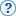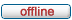# forum.alglib.net

ALGLIB forum
 It is currently Sun Sep 25, 2022 2:21 pm

 All times are UTC

### Forum rules

1. This forum can be used for discussion of both ALGLIB-related and general numerical analysis questions
2. This forum is English-only - postings in other languages will be removed.

 Print view Previous topic | Next topic
Author MessagePost subject: problem applying RBF example rbf_d_ml_simple examplePosted: Thu May 02, 2013 6:53 amJoined: Tue Apr 23, 2013 3:18 am
Posts: 1
It maybe silly but I read over the example "rbf_d_ml_simple example" and found me not pro enough at statistics to realize the algorithm of RBF code T_T

I intended to write a method which could convert grid data into different intervals for GIS performance with RBF functions. But tested with ecmwf temperature grid data?value of which is between -20 to 40?I got values larger than 100...

Were the parameters set wrong especially 'lambda' of rbfsetalgomultilayer?

The function is written as below:

Code:
int interpolationmetod1()
{
//
// This example shows how to build models with RBF-ML algorithm. Below
// we assume that you already know basic concepts shown in the example
// on RBF-QNN algorithm.
//
// RBF-ML is a multilayer RBF algorithm, which fits a sequence of models
// with decreasing radii. Each model is fitted with fixed number of
// iterations of linear solver. First layers give only inexact approximation
// of the target function, because RBF problems with large radii are
// ill-conditioned. However, as we add more and more layers with smaller
// and smaller radii, we get better conditioned systems - and more precise models.
//
rbfmodel model;
rbfreport rep;
double v;
int lon=361,lat=281;

//
// We have 2-dimensional space and very simple interpolation problem - all
// points are distinct and located at straight line. We want to solve it
// with RBF-ML algorithm. This problem is very simple, and RBF-QNN will
// solve it too, but we want to evaluate RBF-ML and to start from the simple
// problem.
//     X        Y
//     -2       1
//     -1       0
//      0       1
//     +1      -1
//     +2       1
//
rbfcreate(2, 1, model);
real_2d_array xy0;
xy0.setlength(lat,lon);
qDebug()<<"matrix done";
//
//read data of diamond4 type
//
QString str,filename="E:\\13042420.003";
QFile in(filename);
{
qDebug()<<filename<<" not exists";
return 0;
}
QTextStream file(&in);
QFile out("e:\\interpolation.txt");
out.open(QIODevice::WriteOnly);
QTextStream ts(&out);

int steplon=0, steplat=0;
//qDebug()<<"pos1:"<<sd.toString("yyyyMMdd");
while(!file.atEnd())
{
file>>str;
xy0(steplat,steplon)=str.toDouble();
//ts<<"("<<steplat<<","<<steplon<<") "<<str<<"="<<xy0(steplat,steplon)<<" the same?"<<endl;
if(steplon>=(lon-1))
{
steplon=0;
steplat++;
}
else
steplon++;
if(steplat==281)
break;
}
in.close();

rbfsetpoints(model, xy0);

//
// First, we try to use R=5.0 with single layer (NLayers=1) and moderate amount
// of regularization.... but results are disappointing: Model(x=0,y=0)=-0.02,
// and we need 1.0 at (x,y)=(0,0). Why?
//
// Because first layer gives very smooth and imprecise approximation of the
// function. Average distance between points is 1.0, and R=5.0 is too large
// to give us flexible model. It can give smoothness, but can't give precision.
// So we need more layers with smaller radii.
//
//rbfsetalgomultilayer(model, 5.0, 1, 1.0e-3);
//rbfbuildmodel(model, rep);
//printf("%d\n", int(rep.terminationtype)); // EXPECTED: 1
//v = rbfcalc2(model, 0.0, 0.0);
//printf("%.2f\n", double(v)); // EXPECTED: -0.021690

// Now we know that single layer is not enough. We still want to start with
// R=5.0 because it has good smoothness properties, but we will add more layers,
// each with R[i+1]=R[i]/2. We think that 4 layers is enough, because last layer
// will have R = 5.0/2^3 = 5/8 ~ 0.63, which is smaller than the average distance
// between points. And it works!
rbfsetalgomultilayer(model, 5.0, 4, 1.0e-2);
rbfbuildmodel(model, rep);
printf("%d\n", int(rep.terminationtype)); // EXPECTED: 1
ts<<"now start interpolation"<<endl;
for(double stepy=0; stepy<lat;stepy+=0.2)
{
for(double stepx=0; stepx<lon;stepx+=0.2)
{
//ts<<xy0(stepy,stepx)<<" ";
v = rbfcalc2(model, stepy, stepx);
ts <<"("<<stepy<<","<<stepx<<") = "<< v<<" ";
if((stepx-floor(stepx)<0.001)&&(stepy-floor(stepy)<0.01))
ts<<xy0(stepy,stepx);
ts <<endl;
}
//ts<<endl;
}
out.close();
//printf("%.2f\n", double(v)); // EXPECTED: 1.000000

// BTW, if you look at v, you will see that it is equal to 0.9999999997, not to 1.
// This small error can be fixed by adding one more layer.
return 0;
}

Hope someone could help me, thanks.

TopDisplay posts from previous: All posts1 day7 days2 weeks1 month3 months6 months1 year Sort by AuthorPost timeSubject AscendingDescending

 All times are UTC

#### Who is online

Users browsing this forum: Bing [Bot] and 1 guest

 You cannot post new topics in this forumYou cannot reply to topics in this forumYou cannot edit your posts in this forumYou cannot delete your posts in this forumYou cannot post attachments in this forum

Search for:
 Jump to:  Select a forum ------------------ ALGLIB forum    ALGLIB-discussPowered by phpBB © 2000, 2002, 2005, 2007 phpBB Group